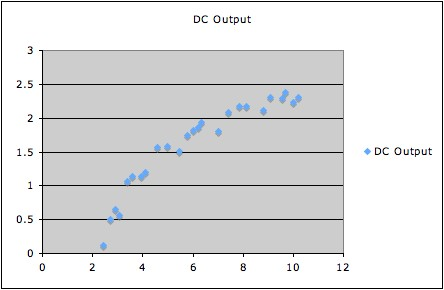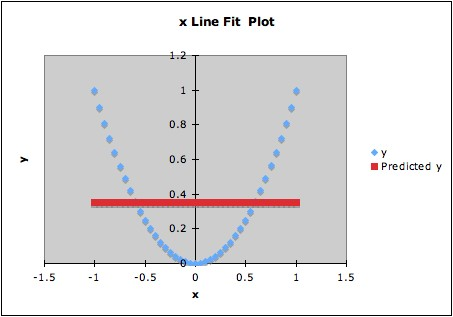# Misinterpreting the Overall F-Statistic in Regression

Most software includes an "overall F-statistic" and its corresponding p-value in the output for a least squares regression. This is the statistic for the hypothesis test with null hypothesis

H0: All non-constant coefficients in the regression equation are zero

and alternate hypothesis

Ha: At least one of the non-constant coefficients in the regression equation is non-zero.

More explicitly, if Y is the response variable and the predictors are X1, X2, ... , Xm and the model equation1 assumed for the regression is

(*) E(Y|X1, X2, ... , Xm) = β0 + β1 X1+ β2 X2+ ... + βmXm

then the null and alternate hypotheses for this F-test are

H0: β1 = β2 = ... = βm= 0
and
Ha: At least one of β1, β2 , ... or βm is non-zero.

Misinterpreting the output for this hypothesis test is a common mistake in regression. Two types of mistakes are common here.

First type of mistake: Assuming that if the output for this hypothesis test has a small p-value, then the regression equation fits the data well.

Second type of mistake: Assuming that if the output for this hypothesis test does not show statistical significance, then Y does not depend on the variables X1, X2, ... , Xm.

Both mistakes are based on neglecting a model assumption -- namely, the assumption expressed by (*): that the conditional mean E(Y|X1, X2, ... , Xm) is a linear function of the variables X1, X2, ... , Xm.

Examples of each type of mistake:

1. The following graph shows DC output vs. wind speed for a windmill.Running a regression with model assumption

E(DC output|wind speed) = β0 + β1×(wind speed)

gives overall F-statistic 160.257 with 1 degree of freedom, and corresponding p-value 7.5455E-12, which is certainly statistically significant.

However, the data clearly have a curved pattern; thus a model equation expressing a suitable curved relationship will fit better than a linear model equation. (For a good way to do this, see Example 3 of Overinterpreting High R2.) All that the F-statistic says is that we have strong evidence that the best fitting line has non-zero slope (which is pretty clear from the picture anyhow).

Of course, in a case with several predictor variables, it is typically difficult (if not impossible) to tell in advance whether or not a linear model fits. Thus, unless there is other evidence that a linear model does fit, all that a statistically significant F-test can say is that the data give evidence that the best-fitting linear model of the type specified has at least one predictor with a non-zero coefficient.

One method that sometimes works to get around this problem is to (attempt to) transform the variables to have a multivariate normal distribution, then work with the transformed variables. This will ensure that the conditional means are a linear function of the transformed explanatory variables, no matter which subset of explanatory variables is chosen. Such a transformation is sometimes possible with some variant of a Box-Cox transformation procedure. See, e.g., pp. 236 and 324 - 329 of Cook and Weisberg's text2 for more details.

2. The following graph shows data and the computed regression line.The fitted regression line is y = 0.35 + 0x. The overall F-statistic is essentially 0, giving p-statistic essentially 1. However, the data are constructed so that y depends on x: y = x2. Thus there is a strong dependence of y on x, but the F-test for the linear model does not detect this at all.

Notes:

1.  In the expresion used above, E(Y|X1, X2, ... , Xm) refers to the mean of the conditional distribution of Y given X1, X2, ... , Xm; see also Overfitting. Depending on notation used, the model equation might be expressed in different ways, for example as

Y = β0 + β1 X1+ β2 X2+ ... + βmXm + ε
or as
yi = β0 + β1 xi1+ β2 xi2+ ... + βmxim + εi

2. Cook and Weisberg (1999) Applied Regression Including Computing and Graphics, Wiley.

Last updated June 13, 2014